# A book tossed in the air

Here, we explore the behavior of a book tossed in the air by developing a model that governs the book’s motion. Through simulation and animation, we wish to confirm the well-known result that rotation about the book’s intermediate principal axis is unstable in the face of rotational perturbations; in contrast, the spin behavior about the book’s other two principal axes is stable. This topic is discussed in various textbooks, such as  and . A related scenario involves tossing a tennis racket into the air while giving it an initial angular velocity about an axis parallel to the racket’s face, which causes the racket to flip through 180. This phenomenon is explained in  and can be observed in the video in Figure 1.

Contents

## Equations of motion

Referring to Figure 2, we represent the book by a rigid and uniform rectangular prism of mass, length, width, and thickness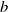. The book’s reference configuration is defined such that the book’s mass center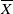is located at the origin and the space-fixed basis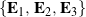is aligned with the book’s principal axes. We locate the mass centerrelative to the origin using a set of Cartesian coordinates,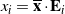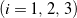. The book’s corotational basisis related to the fixed basis by a rotation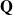such that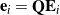.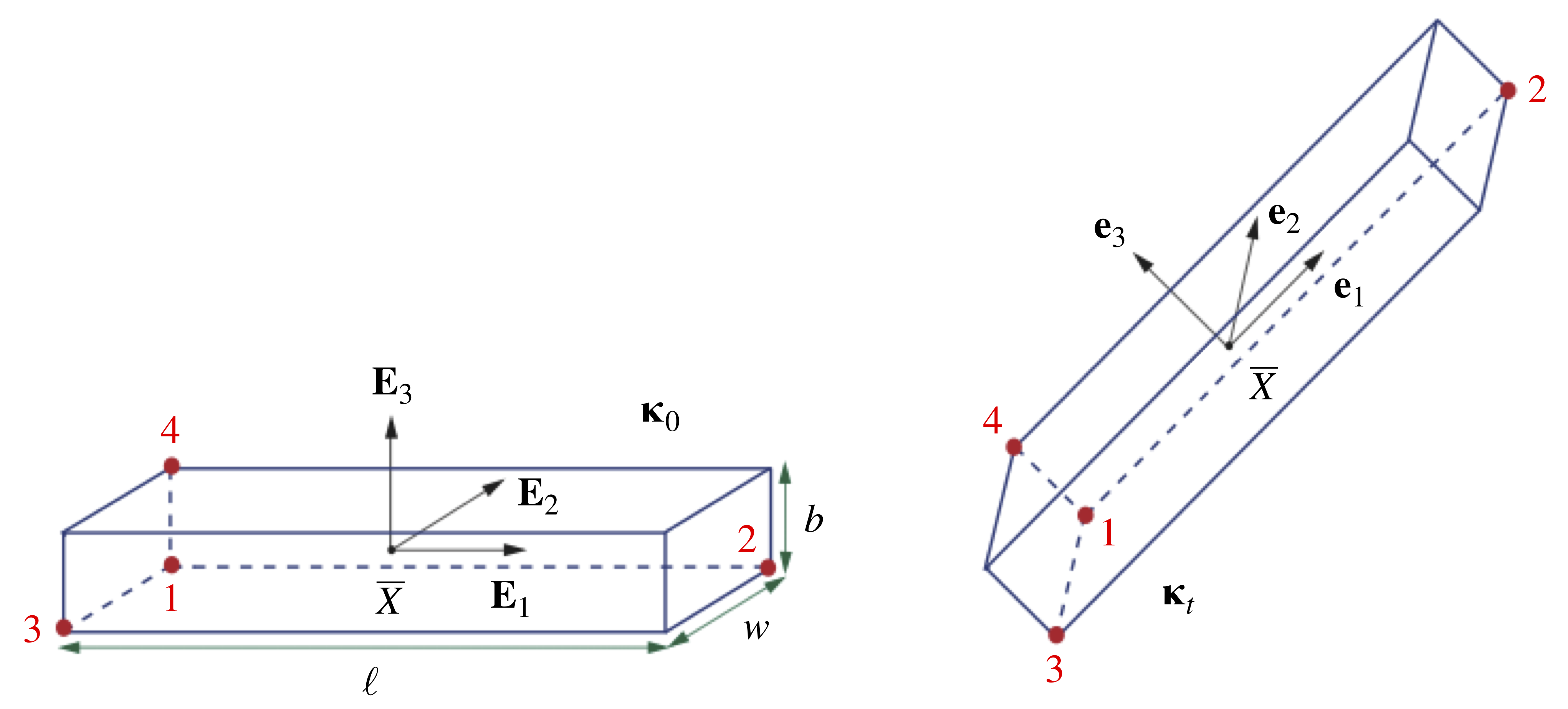Figure 2. Schematic of a book, represented by a rectangular prism, in the reference and current configurations, and , respectively. The points labeled 1 through 4 are provided for perspective.

We can obtain the equations of motion governing the translation of the mass centerand the book’s orientation by applying balances of linear and angular momenta, respectively. Neglecting the effect of air drag, the only force exerted on the book after being tossed into the air is the weight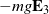acting at the center of mass, and so a balance of linear momentum yields the system of equations

(1)while a balance of angular momentum with respect to the mass center gives

(2)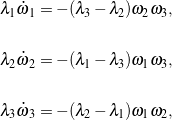where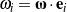are the corotational components of the book’s angular velocity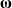, and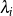are the principal moments of inertia:

(3)Suppose we use a set of 3-2-1 Euler angles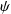,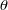, and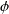to parameterize the rotation tensor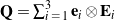. In this case, the tensor’s components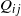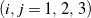, when arranged in a matrix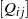, have the representation

(4)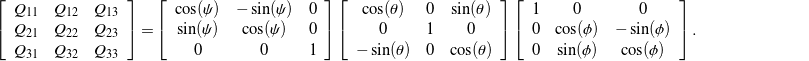Additionally, the corotational angular velocity components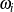are related to the Euler angles,, andand their rates of change by

(5)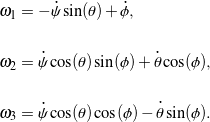Lastly, by introducing the state vector

(6)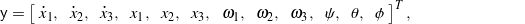we can express the the governing equations in (1), (2), and (5) for the translation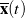of the book’s mass center and the book’s orientationin the first-order matrix-vector formfor numerical integration in MATLAB, where

(7)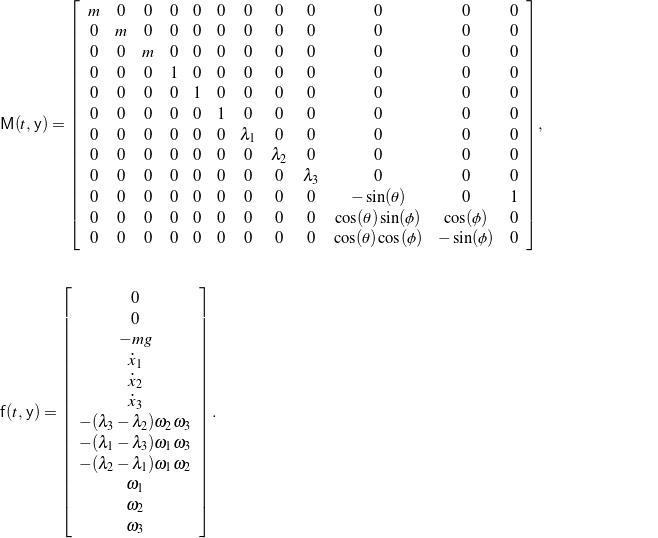## Simulation and animation

In simulating the book’s behavior when tossed in the air, we take the book to initially be in an unrotated state with its mass center at the origin: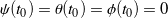and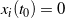. The book is launched vertically, in which case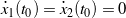and, and an initial spin is imparted to examine the stability of rotation about each principal axis. Suppose we first toss the book with an initial spin rate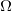about its longitudinal axis (i.e., its first principal axis) and with small perturbationsabout the other two principal axes:and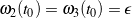. As shown in the animation in Figure 3, the resulting motion, using values of the physical parameters representative of a small textbook, indicates that the tossed book spins stably about this axis. One corner of the book is highlighted with a blue marker to help better visualize the rotation.

Next, launch the book with a spin largely about its third principal axis, which corresponds to the axis perpendicular to the book’s front and rear covers: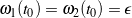and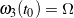. The animated response in Figure 4 demonstrates that rotation about this axis is also stable.

Lastly, impart a spin mostly about the book’s intermediate (i.e., second) principal axis such that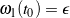,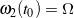, and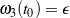. As expected, and similar to the tennis racket behavior shown in Figure 1, we see from the animation in Figure 5 that the book tumbles in the air instead of stably spinning about this axis.

For convenience, an interactive, web-based JavaScript simulation that demonstrates the same behavior animated in Figures 35 is accessible through Figure 6.Figure 6. Screenshot of a JavaScript-based book-tossing simulation. Clicking on the above image will open the simulation in a new browser tab; Google Chrome is recommended. We are grateful to Tung Phan for creating this simulation.